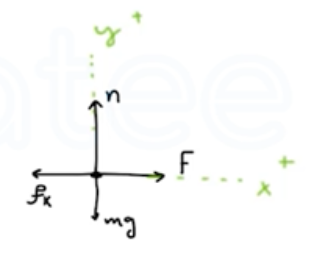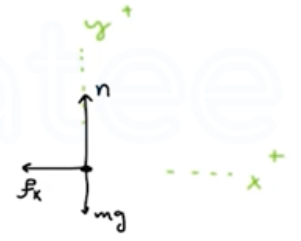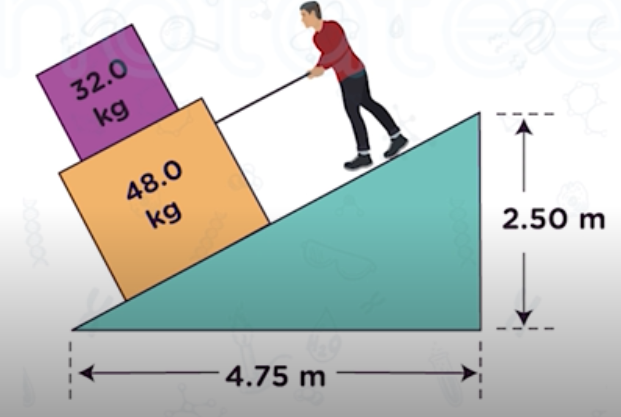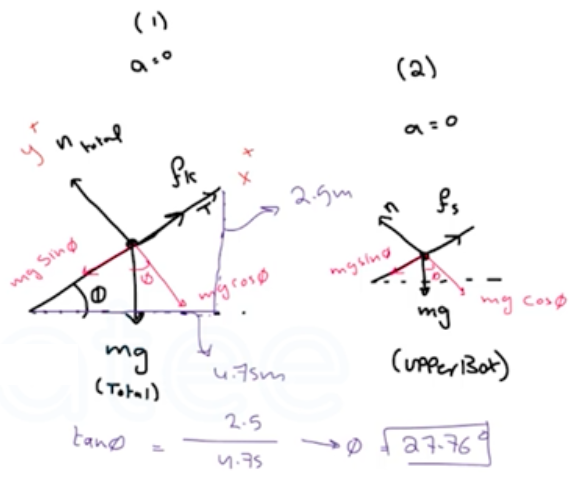Need Help?

Subscribe to Physics 1

###### \${selected_topic_name}
• Notes

$\begin{array}{l}{\text { A stockroom worker pushes a box with mass } 11.2 \mathrm{kg}} \\ {\text { on a horizontal surface with a constant speed of } 3.50 \mathrm{m} / \mathrm{s} \text { . The }} \\ {\text { coefficient of kinetic friction between the box and the surface is }} \\ {0.20 . \text { (a) What horizontal force must the worker apply to maintain }} \\ {\text { the motion? (b) If the force calculated in part (a) is removed, how }} \\ {\text { far does the box slide before coming to rest? }}\end{array}$

$m=11.2 \mathrm{kg}$

$V=3.5 \mathrm{m} / \mathrm{s} \rightarrow a=0$

$\mu_{k}=0.2$$\sum F_{y}=m a_y$

$n-m g=0 \rightarrow n=m g$

$\sum F_{x}=ma_{ x}$

$F-f_{k}=0 \rightarrow F=f_{k}=\mu_{k} m g=0.2*1 1.2 * 9.8$

$=22 \mathrm{N}$

$m=11.2 \mathrm{kg}$

$V_{0}=3.5 \mathrm{m} / \mathrm{s} \rightarrow a=0$

$\mu_{k}=0.2$

$V=0$$\sum F_{x}=m a_ x \rightarrow-f_{k}=m a_ x \rightarrow -\mu_{k} mg=ma_{{x}}$

$∴ a_ x=-\mu_{k} g=-(0.2)(9.8)=-1.96 \mathrm{m} / \mathrm{s}^{2}$

$V x^{2}=V_{0 x}^{2}+2 a_{x}\left(x-x_{0}\right) \rightarrow\left(x-x_{0}\right)=\frac{V_ x^{2}-V_{0x}^{2}}{2 a_ x}$

$\rightarrow {x-x_0}=\frac{0-(3.5)^{2}}{2(-1.96)}=3.1 \mathrm{m}$

$\begin{array}{l}{\text { You are lowering two boxes, one on top of the other, }} \\ {\text { down the ramp shown in Fig. E5.31 by pulling on a rope parallel to }} \\ {\text { the surface of the ramp. Both boxes move together at a constant }} \\ {\text { speed of } 15.0 \mathrm{cm} / \mathrm{s} \text { . The coefficient of kinetic friction between the }} \\ {\text { ramp and the lower box is } 0.444, \text { and the coefficient of static fric- }} \\ {\text { tion between the two boxes is } 0.800 . \text { (a) What force do you need to }} \\ {\text { exert to accomplish this? (b) What are the magnitude and direction }} \\ {\text { of the friction force on the upper box? }}\end{array}$$\sum F_ y=m a_y$

$n_{total}-mg \cos \theta=0$

$\rightarrow n_{total}=m_{total} g \cos \theta$

$=(32+48)(9.8) \cos (27.76)$

$=693.8 N$

$\sum F_{x}=ma_ x \rightarrow f_{k}-M_{total } g \sin \theta+T=0$

$f_{k}+T-m_{tot}g \sin \theta=0$

$\mu_{k} m_{tot} g \cos \theta+T-m_{tot}g \sin \theta=0$

$\rightarrow T=m_{tot}g\left(\sin \theta-\mu_{k} \cos \theta\right)=(80)(9.8)[\sin 27.76-0.444 \cos 27.76]$

$∴ T=57.13 \mathrm{N}$

$\sum F_{x}=ma_x \rightarrow f_s-mg \sin \theta=0 \rightarrow f_s=mg \sin \theta$

$\rightarrow f_{s}=(32)(9.8) \sin (27.76)=146 \mathrm{N}$